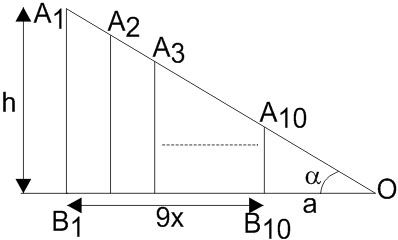#### Let 10 vertical poles standing at equal distances on a straight line, subtend the same angle of elevationat a point O on this line and all the poles are on the same side of O. If the height of the longest pole is ‘h’ and the distance of the foot of the smallest pole from O is ‘a’ ; then the distance between two consecutive poles, is : Option 1)Option 2)Option 3)Option 4)As we learnt in

Height and Distances -

The height or length of an object or the distance between two distant objects can be determined with the help of trigonometric ratios.

-

For all poles to subtend the same angle, the longest pole must be farthest, smallest pole must be nearest, while all other poles are arranged in ascending order of height. Let distance between each pole be x. Then,In,..............( 1 )

After solving, we get:Option 1)This option is incorrect.

Option 2)This option is incorrect.

Option 3)This option is correct.

Option 4)This option is incorrect.

#### Sabhrant Ambastha